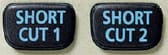# FC-100V | CASIO

Search

AAA-size battery
Financial Calculators

# FC-100V

My GShock

## FeaturesPlastic keys

Comes with new slide-on hard case

Power supply:AAA × 1 (R03)

Approximate battery life:2 years

Dimensions:13.7(H) × 80(W) × 161(D) mm

Approximate weight:110g

## Direct mode key

A bank of mode keys provides you with one-touch access to the mode you need.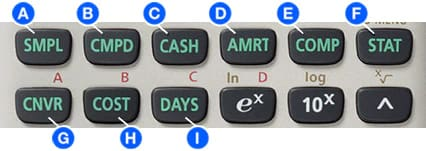Simple interest

Interest amount, principal and interest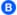Compound interest

Payment period, interest rate, deposit amount, future value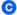Investment Appraisal (cash flow)

Net present value method, internal rate of return method, payback period method, etc.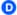Amortization

Monthly payment, principal and interest to date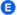General and function

Virtually the same functions as a standard calculator.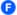Statistical and regression

Statistical calculations using input sample data.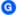Interest rate conversion

Nominal interest rate and effective interest rate conversion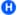Cost, selling price,or margin

Calculation of any of the above values after inputting the other two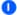Day or date calculations

Virtually the same as a standard calculator, with some variation in the input method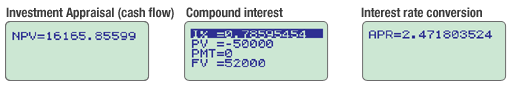FC-200V FC-100V
Simple interestCompound interestInvestment Appraisal (cash flow)AmortizationInterest rate conversionCost,selling price,or marginDay or date calculationsDepreciationBond calculationBreak-even pointGeneral and FunctionStatistical and regression## Easy operation with parameters

A full-dot 4-line display provides easier scrolling between parameters and simplifies input, confirmation, and editing.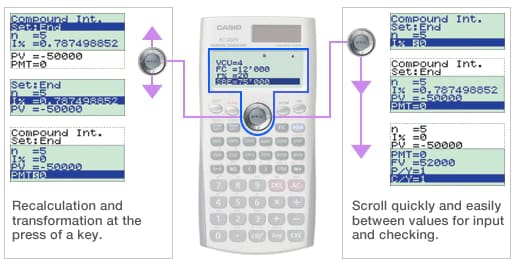## Calculate the result.

The result appears immediately after you press the SOLVE key.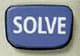## Create shortcuts.

Once you use a parameter value or setting in a calculation, you can assign it to a shortcut key for instant recall whenever you need it. This feature is great for repeat calculations.